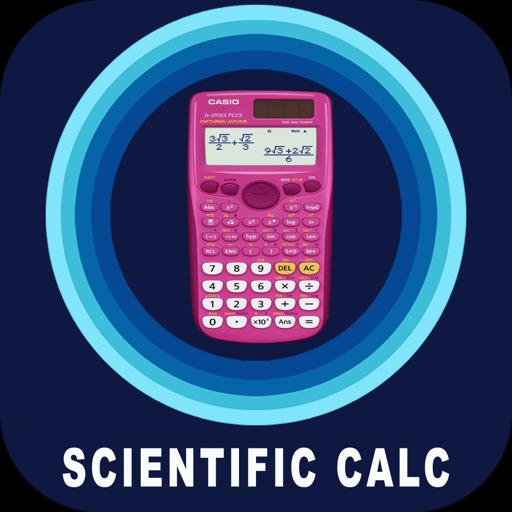## Multi purpose Scientific Calculator App helps in carrying out advanced mathematical calculations & operations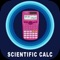# Real Scientific Calculator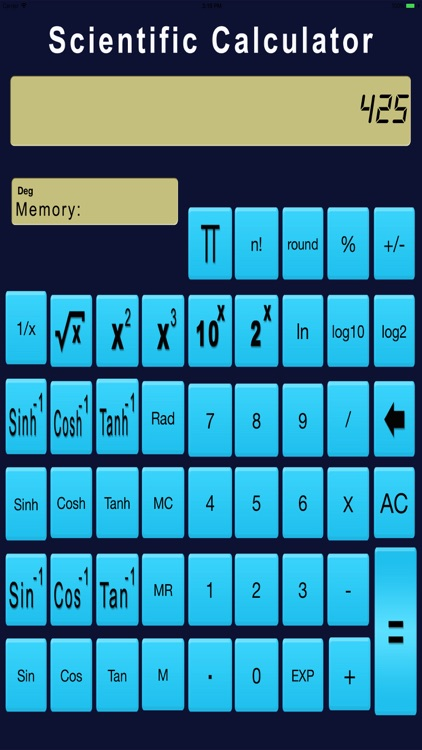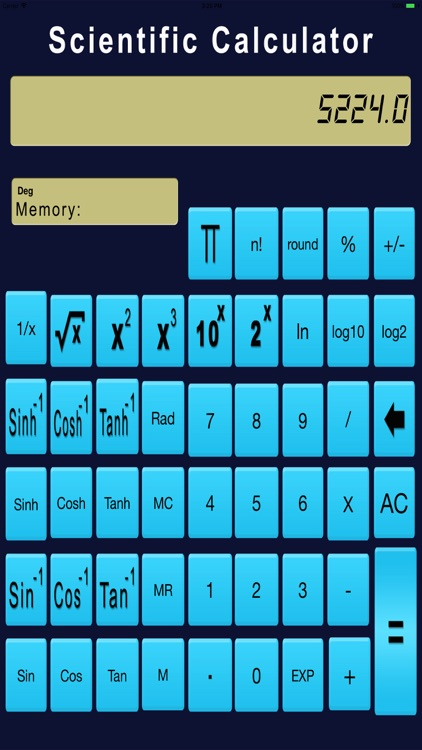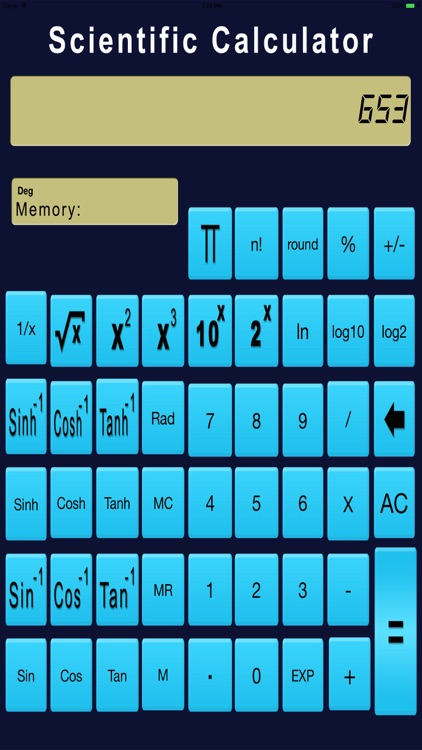Multi purpose Scientific Calculator App helps in carrying out advanced mathematical calculations & operations.### App Details

Version
1.0
Rating
NA
Size
18Mb
Genre
Finance Utilities
Last updated
December 23, 2017
Release date
December 23, 2017

### App Screenshots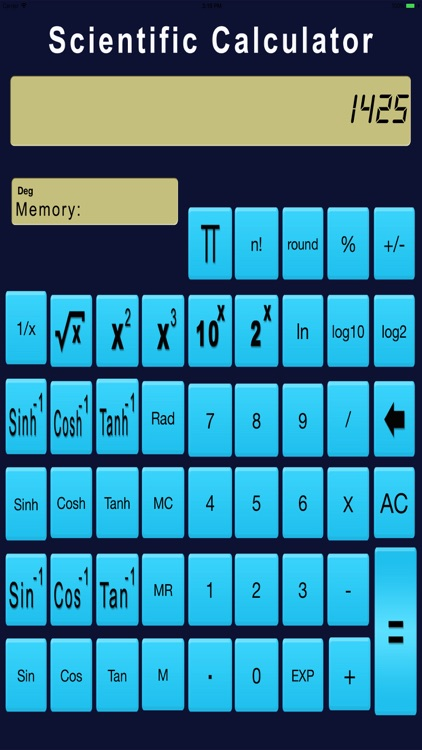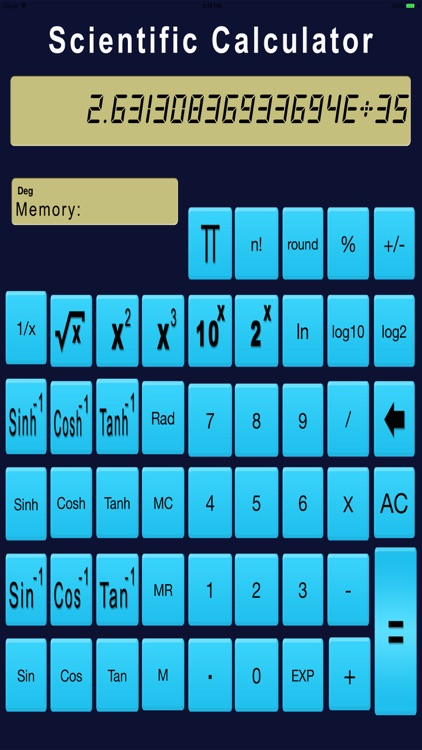### App Store Description

Multi purpose Scientific Calculator App helps in carrying out advanced mathematical calculations & operations.

Operations available:
Square root, cube root
Square power, cube power
Trigonometric functions
Permutation, combination
Percentage calculation
Fraction conversion
Supports binary, octal and hexadecimal calculations
Degree,Minute,Second Calculations